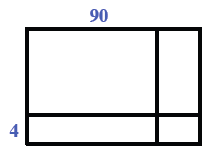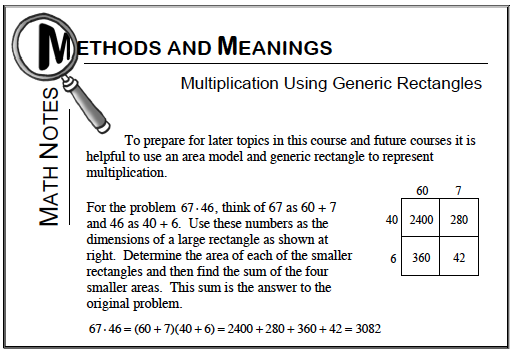### Home > CC1 > Chapter 5 > Lesson 5.3.1 > Problem5-75

5-75.

Draw generic rectangles to calculate each of the following products. Homework Help ✎

1. $34 \cdot 91$

• Refer to the Math Notes box below. The generic rectangle below has been started for you.

••1. $421 \cdot 36$

Refer to the previous part for help. How will the $3$-digit number $(421)$ make this rectangle look different?